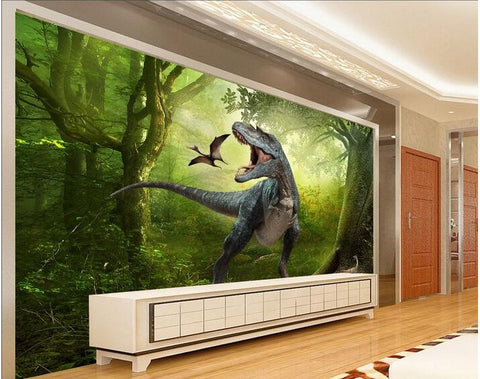## 3D T-Rex Dinosaur Forest Wall Mural Jurassic Photo Wallpaper for Walls

• \$39.95

Product Details

• Function: Waterproof, Smoke-Proof, Moisture-Proof, Fireproof, Mold Proof, Soundproof, Sound-Absorbing, Heat Insulation, Anti-static
• Material: Non-woven
• Pattern:  3D T-Rex Dinosaur

IMPORTANT: PLEASE READ\$39.95 is for 1 piece. only.  It is very small, about 4'7" x 2'4". To cover a complete wall you will need more pieces.  The example pictures show a wall that is fully covered with wallpaper.  You need to measure your wall and calculate how many pieces you need.  If you need help, please contact us.  If not, just follow these steps:

1. Click "add to cart". It will take you to checkout.

2. Select the number you wish to purchase from the drop down menu. Total will be calculated for you.

*How to calculate how many pieces you need:
1). Measure your wall.  Convert your measurements to centimeters (you can use any online metric converter). 2). If your wall is 360cm width x 240cm height for example, multiply those numbers: 360 x 240 = 8.6.  So you would need to buy 9 pieces.

Here are some fixed sizes:

Quantity 1  :  1 square meter = 140cm(W) x 70cm(H) (4'7" x 2'4") \$39.95

Quantity 2  :  2 square meter = 200cm(W) x 100cm(H) (6'7" x 3'3") \$79.90

Quantity 3  :  3 square meter = 220cm(W) x 140cm(H) (7'3" x 4'7") \$119.85

Quantity 4  :  4 square meter = 250cm(W) x 160cm(H) (8'2" x 5'3") \$159.80

Quantity 5  :  5 square meter = 280cm(W) x 180cm(H) (9'2" x 5'11") \$199.75

Quantity 6  :  6 square meter = 300cm(W) x 200cm(H) (9'10" x 6'7") \$239.70

Quantity 7  :  7 square meter = 330cm(W) x 210cm(H) (10'10" x 6'11") \$279.65

Quantity 8  :  8 square meter = 360cm(W) x 230cm(H) (11'10" x 7'6") \$319.60

Quantity 9  :  9 square meter = 380cm(W) x 240cm(H) (12'5" x 7'10") \$359.55

Quantity 10 :  10 square meter = 400cm(W) x 250cm(H) (13'1" x 8'2") \$399.50

Quantity 11 : 11 square meter = 420cm(W) x 260cm(H) (13'9'' x 8'6'') \$439.45

Quantity 12 : 12 square meter = 440cm(W) x 270cm(H) (14'5" x 8'10") \$479.40

Quantity 13 : 13 square meter = 460cm(W) x 280cm(H) (15'1'' x 9'2'') \$519.35

Quantity 14 : 14 square meter = 480cm(W) x 290cm(H) (15'9'' x 9'6'') \$559.30

Quantity 15 : 15 square meter= 500cm(W) x 300cm(H) (16'5'' x 9'10'') \$599.25

Quantity 16 : 16 square meter=500cm(W) x 320cm(H) (16'5" x 10'6") \$639.20

We Also Recommend